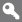X

to continue to ilmkidunya.com

X

to continue to ilmkidunya.com

X

### Register Type

Please Provide following information to Register

•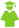Student
•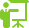Tutor
•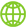Consultant
•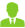Employer

# CSS Examination Syllabus - Statistics

Statistics ( Total Marks - 100 )

1. Basic Probability Axiomatic definition of probability, random variable, distribution function, probability density function, mathematical expectation; conditional probability, jointly distributed random variables, marginal and conditional distributions, conditional expectation, stochastic independence.
2. Some Special Distributions : Binomial, poisson. negative binomial, hypergeometric, normal distributions with their derivation of their mean and variance; Definition and Application of chisquare, "T" and 'F' distributions.
3. Statistical Inference: Maximum likelihood estimation of the mean and the variance of a normal population; confidence interval for mean, difference of means and for variance: testing hypothesis for the equality of two means (paired and unpaired observations); testing of equality of sever al means (ANOVA) and testing of variance and equality of two variance.
4. Correlation and regression: Simple linear regression model point and interval estimation of parameters, Simple Partial, Multiple Correlation and testing of these correlations.
5. Sampling, Simple random, stratified, systematic and cluster sampling, estimates of mean and total and their precision.
6. Applications of Statistics in social, economic and political problems public health, crimes, Law, social innovations economic development, socio-political inequality.

• Title
• Author
• 1
• Introduction to the Theory of Statistics
• Mood, Graybill and Boes
• 2
• Mathematical Statistics
• Freund
• 3
• Mathematical Statistics
• Hood and Craig
• 4
• Sampling Techniques (3e)
• Cochran and Cox
• 5
• Statistics: An Introductory Analysis
• Yamane
• 6
• Statistics: A Guide to the Unknown
• Tanur; Hudith (ed)

## Check other Subjects and Syllabus below:

#### Compulsory Subjects

General Knowledge

### Optional Subjects:

(subjects carrying a total of 600 marks to be selected)

#### Group A

Subjects carrying not more than 200 marks can be opted.

#### Group B

Subject/s carrying not more than 200 marks can be opted.

#### Group C

Subjects carrying not more than 200 marks can be opted.

#### Group D (Science Subjects )

Subject/s carrying not more than 200 marks can be opted.

#### Group E (History Subjects )

Subjects carrying not more than 200 marks can be opted.

#### Group F

Subject/s carrying not more than 200 marks can be opted.
Note: International Law CANNOT BE opted in combination with International Relations.

#### Group G

Subjects carrying not more than 200 marks can be opted.

#### Group H (Regional Languages )

Subject/s carrying not more than 200 marks can be opted.
Regional Languages: Not more than one of the 4 subjects can be opted.

#### Group I (National and Foreign Languages )

Subjects carrying not more than 200 marks can be opted.
National/Foreign/Classical Languages: Not more than one of 4 subjects can be opted. Note: Urdu can not be combined with Regional Languages.